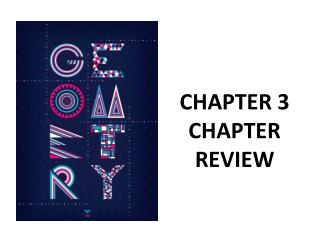DownloadDownload PresentationCHAPTER 3 CHAPTER REVIEW

# CHAPTER 3 CHAPTER REVIEW

Télécharger la présentation## CHAPTER 3 CHAPTER REVIEW

- - - - - - - - - - - - - - - - - - - - - - - - - - - E N D - - - - - - - - - - - - - - - - - - - - - - - - - - -
##### Presentation Transcript

1. CHAPTER 3 CHAPTER REVIEW

2. Relationships Between Lines: GOAL: Identify relationships between lines Two lines are parallel lines if they lie in the same plane and do not intersect. Two lines are perpendicular lines if they intersect to form a right angle. p m n q

3. Two lines are skew lines if they do not lie in the same plane. Skew Lines never intersect. c b ● A

4. All segments in the diagram are part of a line and all corners of the cube form right angles. R V Name a line that is skew to VW. Name a plane that appears parallel to plane VWX. Name a line that is perpendicular to plane VWX. Q U W S T X

5. THEOREMS ABOUT PERPENDICULAR LINES: GOAL: Use theorems about perpendicular lines Theorem 3.1 Words: All right angles are congruent. A Symbols: If the m A = 90° and m B = 90°, then A B B

6. Theorem 3.2 Words: If two lines are perpendicular, then they intersect to form four right angles. Symbols: If n m, then m 1 = 90°, m 2 = 90°, m 3 = 90°, and m 4 = 90°. 1 4 2 3

7. Theorem 3.3 Words: If two lines intersect to form adjacent congruent angles, then the lines are perpendicular. ● B A 1 2 ● D ● C Symbols: If 1 2, then AC BD

8. Theorem 3.4 Words: If two sides of adjacent acute angles are perpendicular, then the angles are complementary. F ● ● G 3 4 ● H ● E Symbols: If EF EH, then m 3 + m 4 = 90°

9. In the diagram at the right, EF EH and m GEH = 30 °. Find the value of y. F ● 2y – 12 E ● 30° ● G ● H

10. Name a pair of alternate interior angles Name a pair of corresponding angles. Name a pair of same-side interior angles. Name a pair of alternate exterior angles. 1 3 2 4 5 7 6 8

11. If j ǁ k then find: m<1 m<2 m<3 m<4 m<5 m<6 m<7 j 72° 1 2 3 k 4 5 6 7

12. 120° 2x + 32 Solve for x.

13. 45° 3x – 15 Solve for x.

14. 74° 5x + 14 Solve for x.

15. 103° 6y – 23 Solve for y.

16. Showing Lines are Parallel Goal: Show that two lines are parallel. The converse of an if-then statement is the statement formed by switching the hypothesis and the conclusion. Write the converse for the given if-then statement: 1. If two angles have the same measure, then the two angles are congruent.

17. Examples: Determine the postulate that proves the lines are parallel. 63° 2. 1. 55° 125° 63°

18. 138° 3. 4. 56° 138° 56° 145° 5. 145°

19. Theorem 3.11 Words: If two lines are parallel to the same line, then they are parallel to each other. Symbols: If q ǁ r and r ǁ s, then q ǁ s. q r s

20. Theorem 3.12 Words: In a plane, if two lines are perpendicular to the same line, then they are parallel to each other. Symbols: If m p and n p then m ǁ n. n m p

21. PRACTICE PAGE 160-163 1-32